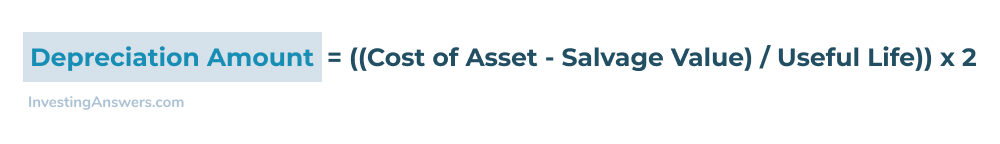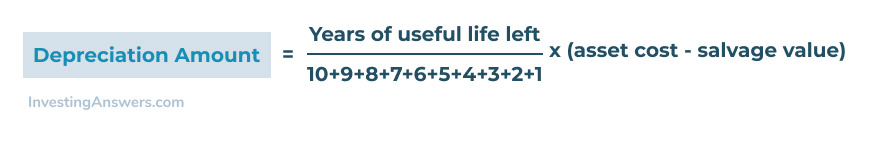## What is Accelerated Depreciation?

Accelerated Depreciation is a form of depreciation used for accounting or tax purposes to reduce the book value of an asset faster than traditional straight-line depreciation.

This allows businesses to deduct more depreciation in the early years of an asset to help reduce taxable income, which in turn helps increase after-tax cash flow which can be reinvested into the business.

## How does Accelerated Depreciation Work?

Depreciation of any kind is a reduction in the value of an asset that is placed in service for business purposes. The wear, tear, and usage of that asset will lower its value over time, and for accounting purposes, this is an expense to the business owner.

If you calculate the reduction in the value of an asset by dividing its value by its useful life (in years), this is called straight-line depreciation. This method assumes that the asset will lose value evenly over its lifespan.

For example, with straight-line depreciation, if you pay \$20,000 for a truck and you expect it to have value for 10 years, your depreciation expense is \$2,000 per year.

Accelerated depreciation assumes that the value of an asset will lower more quickly in the first few years in service (due to high use). It was also created as a way for business owners to lower taxable income while investing in a business by purchasing assets.

## Types of Accelerated Depreciation

There are multiple ways to calculate accelerated depreciation. In fact, there are two separate categories, each with their own set of options.

The first category is for GAAP -- which stands for 'generally accepted accounting principals. This is the accounting method that public companies use to put together their financial statements.

The second category is for tax purposes and is calculated separately from GAAP. GAAP methods do not impact what is paid in taxes.

Here's how each of them works:

## GAAP Methods

These methods are used for accounting purposes but not tax purposes.

### Double Declining Balance Method (DDB)

With the double declining balance method, the asset is depreciated twice as fast as under the straight-line depreciation method. This is calculated by taking the cost of the asset, minus its salvage value, and dividing it by its useful life. This is then multiplied by two to speed up the depreciation process.

The formula for calculating the DDB looks like this:This helps businesses depreciate an asset twice as fast as normal straight-line depreciation.

### Double Declining Balance Method (DDB) Example

A farm purchases a tractor for \$50,000 with a 5-year useful life, and a salvage value (after depreciation) of \$5,000. To accelerate the depreciation, the DDB method is used.

((\$50,000 - \$5,000) / 5 years)) x 2 = \$18,000

Using the DDB formula, the farm will depreciate the tractor by \$18,000 in the first year, instead of \$9,000. Each subsequent year the asset will depreciate by 40% until the salvage value is reached.

Here is how the depreciation is calculated over the tractor's useful life:

 End of Year Book Value Depreciation expense Accumulated depreciation 0 \$50,000 \$0 \$0 1 \$32,000 \$18,000 \$18,000 2 \$19,200 \$12,800 \$30,800 3 \$11,520 \$7,860 \$38,660 4 \$6,912 \$4,608 \$43,268 5 \$5,000 \$1,912 \$45,000

As you can see, the salvage value is reached in year three, fully depreciating the asset much quicker than standard straight-line depreciation.

### Sum of the Year's Digits (SYD)

Another popular method for calculating accelerated depreciation is the sum of the year's digits method.

This method takes the use years left on an asset and divides by the sum of an asset's depreciable years. Here is the formula for calculating the SYD method on a 10-year asset:This formula allows an asset to depreciate more of the asset's value at the beginning of its life.

### Sum of the Year's Digits (SYD) Example

Using the above example of a farm purchasing a tractor with a 10-year use life, here how to use the SYD method for accelerating the depreciation in year one:

((10 / (10+9+8+7+6+5+4+3+2+1)) x (\$50,000 - \$5,000)

In the first year, the depreciation on the tractor would be \$8,181. Each subsequent year would be less until the salvage value is reached in year ten.

## Tax Methods

These methods are used for tax purposes rather than for accounting purposes.

### Section 179

Section 179 of the US Internal Revenue Code allows for an immediate expense deduction of an asset under a certain value. The maximum deduction amount allowed under section 179 is \$1,050,000 on an asset that is up to \$2,620,000 in value (for the year 2021).

This deduction is designed to help companies lower their taxes by allowing the full depreciation of an asset immediately, instead of capitalizing and depreciating the asset over time. This deduction is designed to encourage small business owners to invest in assets for their business.

The deduction is limited to business equipment such as office equipment, machinery, cars and computers. The equipment deducted must be used 50% or more for business purposes.

Using Section 179, the farm would be able to fully depreciate the value of their new tractor in year one, taking the full deduction on their taxes.

### MACRS

MACRS or Modified Accelerated Cost Recovery System is the current US tax depreciation system that companies can use when depreciating their assets. Under this system, companies can recover the capitalized cost of an asset through annual deductions.

MACRS is an accelerated depreciation system that depreciates most of an asset's value in the first few years. There are two systems under MACRS:

• GDS (General Depreciation System)

GDS is the most common depreciation system and it uses a declining balance method (larger deductions in early years of an asset). ADS, on the other hand, depreciates an asset's value over a longer period of time and has smaller yearly deductions than GDS.

To depreciate an asset using MACRS, companies must first determine the asset's classification and which system to use. Once that has been determined, the company must consult IRS Pub 946 to identify the correct depreciation method and depreciation rate for the asset.

## Benefits of using Accelerated Depreciation

Accelerated depreciation can help a business lower its taxable income in the early stages of growth, when income may be lower and asset expenses may be higher. Using an accelerated method of depreciation also offsets some of the costs of business growth and expansion, encouraging business owners to reinvest into the business.

Accelerated depreciation is also a more realistic way to track the value of an asset. A new asset will lose value quicker than a used asset, and therefore accelerated depreciation of that asset is more congruent with its real valuation.

## When to use Accelerated Depreciation

Accelerated depreciation is best used by start-up businesses that need to purchase a large amount of business equipment, but want to offset the cost of that equipment with tax savings. It is also a good idea for businesses that have large equipment expenses to keep up with business growth and expansion. The tax savings can help a business deploy more of its capital on equipment and assets, accelerating business growth.